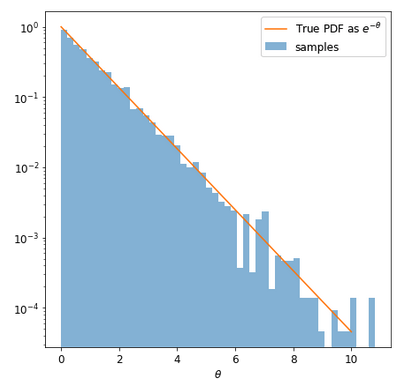# Simple (academic) likelihood for NestedSampler

Hi,
I would like to illustrate NestedSampler(Numpyro wrapper of JaxNS) with a very simple use-case (below delta>0 and \theta>0)

1. As prior an exponential distrib. \pi(\theta) = \delta \exp(-\delta \theta)
2. a Likelihood as L(\theta) = \delta^{-1} \exp(-(1-\delta) \theta)

the posterior is of course post(\theta)=\exp(-\theta) which integral on [0,+infty] is 1. My problem is to setup a valide modeling with the above prior and likelihood.

Is someone can help me?

No idea?

When a consider the problem of adjusting let say a parameter \theta on expermental values (xi,yi) such that y=\theta x, then I proceed like

def my_model(Xspls,Yspls):
theta = numpyro.sample('theta', dist.Normal(0.,10.))
mu = theta*Xspls
sigma = 1.  # here I fix its value for convenience
numpyro.sample('obs', dist.Normal(mu, sigma), obs=Yspls)


Then,

ns = NestedSampler(my_model)
ns.run(random.PRNGKey(2), Xspls=xi, Yspls=yi)
samples = ns.get_samples(random.PRNGKey(3), num_samples=5_000)


works fine.

So, now in my problem the likelihood does not depend on any “external” observation (xi,yi). How I should proceed?

I guess you need numpyro.factor for the likelihood term?

humm, you have an idea to use this factor?

def model():
delta = 0.5
theta = numpyro.sample('theta', dist.Exponential(rate=delta))
factor = - jnp.log(delta) - (1.0-delta)*theta
numpyro.factor("val",factor)


and

ns = NestedSampler(model)
ns.run(random.PRNGKey(0))


The summary crashes:

ns.print_summary()

--------
# likelihood evals: 187098
# samples: 7530
# likelihood evals / sample: 24.8
--------
...
458
459     def _round(v, uncert_v):
--> 460         sig_figs = -int("{:e}".format(uncert_v).split('e')) + 1
461
462         return round(float(v), sig_figs)

IndexError: list index out of range


idea to use this factor?

Your usage is correct. I’m not sure about the error. Probably there is some bugs, instability with the sampler, which returns NaN for logZerr (I don’t know what this is, you can look at ns._results to see all the output of the sampling run).

@fehiepsi

Just to confirm your thought, there are inf… Okay so, I finally got what I was waiting for

def model():
delta = 0.5
theta = numpyro.sample('theta', dist.Exponential(rate=delta))
factor = - jnp.log(delta) - (1.0-delta)*theta
numpyro.factor("val",factor)

ns = NestedSampler(model)
ns.run(random.PRNGKey(0))
data = ns.get_samples(random.PRNGKey(1),100_000)

fig = plt.figure(figsize=(7,7))
plt.hist(data['theta'],bins=50,density=True, alpha=0.5, label='samples');
x_i = np.arange(0,10,0.001)
y_i = np.exp(-x_i)
plt.plot(x_i,y_i,label=r"True PDF as $e^{-\theta}$")
plt.xlabel(r"$\theta$")
plt.show()


One getsand the integral is given by

jnp.exp(ns._results.logZ)


which gives 1.00343113 (the truth is 1) which is ok for N=10^5 samples (ie 1/sqrt(N)).

But JaxNS gives some Inf value for the error, and this is not restricted to my example. It is also the case for JaxNS examples so I get in touch with the author. Anyway thansk for your advise and we can close this thread.

1 Like

Yeah, glad that you found the issue!